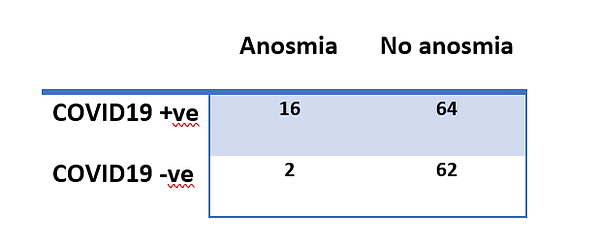top of pageThe relative risk or risk ratio is the ratio of the probability of an outcome in an exposed group to the probability of an outcome in an unexposed group. It is given by:

(A/A+B)/(C/C+D).

In this case it is equal to 6.4

bottom of page